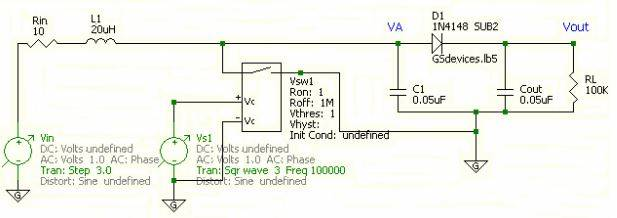# Analysis of a RLC circuit and a Voltage Controlled Switch

• Engineering
Hello everybody,
I am stuck with a circuit that involves voltage controlled switch.

## Homework StatementThe switch is controlled with a square wave generator, Vs1, whose frequency is 100kHz. As far as I know, switch is off when source is on positive phase and vice versa. Therefore, I only need to compute Va when Vs1 is on negative phase, i.e. when the switch is on.
(When it is off, Va is grounded and Vout will decay to zero, however switch goes off before Vout decays. Therefore, me and my friend think that we can assume it to be constant.)

We assume diode is ideal. Then apply Node-Voltage analysis to node VA.

The node between inductor and Rin is called VB and iR is the current on Rin.

## Homework Equations

ic(t)=C$\frac{dVc(t)}{dt}$ ..... (1)
VL(t)=L$\frac{diL(t)}{dt}$ ....(2)

## The Attempt at a Solution

When switch is on (when Va is not grounded),

iR=$\frac{3-VB}{10}$.....(3)

With equations (1) and (3), the following is our Node-Voltage equation,

$\frac{3-VB}{10}$=0.05μ$\frac{dVA}{dt}$+0.05μ$\frac{dVA}{dt}$+$\frac{VA}{100K}$

and from (2) we have,

VB-VA=20μ$\frac{diR}{dt}$→VB=VA+20μ$\frac{d\frac{3-VB}{10}}{dt}$

Is this approach correct?
I mean, if I solve this system of DE, will I obtain the correct functions for node voltages when the switch is on?

Any help is appreciated :)

Last edited:

## Answers and Replies

rude man
Homework Helper
Gold Member
Badly defined problem. When the switch is on, Va is clearly zero from the diagram, contradicting your verbiage. There are other parts of your narrative that confuse.

I would approach the problem with determining the 'big picture' first:

Start with everything discharged and the switch ON, grounding Va. Is there a build up of current? Then, when the switch is OFF, where does the current go and what is the effect on voltage across C1 and Cout? Then, as the number of cycles repeat, how does the voltage Vout build up? To what level? What is the effect of the 100K load? Note that the RL-Cout discharge time cosntant is large compared to the cycle time. Note also that the L1/Rin t.c. is very small.

The time constants tend to be greatly removed from each other, facilitating the analysis of this circuit.

Hello,

By saying "on" I meant switch is open.

Is there a build up of current?

No, all the current go directly to ground when switch is closed.

Then, when the switch is OFF, where does the current go and what is the effect on voltage across C1 and Cout?

The current charges up both capacitors very fast due to small time constant(Rin and 0.05μF) to a maximum voltage value of VA.

Then, as the number of cycles repeat, how does the voltage Vout build up?

Vout is the voltage on Cout. The time constant of Cout when discharging is huge compared to the cycle time as you pointed out Therefore, it decays very little when switch is closed and charges up when it is open.

To what level?

I suppose it is limited to the value of VA?

Update: I simulated this circuit using SPICE. What I said is mostly true, the only part I am wrong is the max value of Vout. How does it get higher than our input which is 3V ? The final voltage at Vout seems to be something between 5 and 6 volts.

Last edited:
rude man
Homework Helper
Gold Member
Hello,

Update: I simulated this circuit using SPICE. What I said is mostly true, the only part I am wrong is the max value of Vout. How does it get higher than our input which is 3V ? The final voltage at Vout seems to be something between 5 and 6 volts.

You can look at your SPICE results to answer that question.

What happens is that eventually the diode stops conducting and Cout is cut off from further charging, leaving only C1 to accept the large inductive currents. Since C1 is half of C1 + Cout, the voltage across C1 eventually doubles compared to the initial voltage across C1 and Cout in parallel, since dv/dt = i/C and C changed from the initial 0.1 uF to the eventual 0.05 uF. The diode stops charging Cout only when Vout has reached Vout = i T/C where T = 5e-6 s and C = Cout = C1.